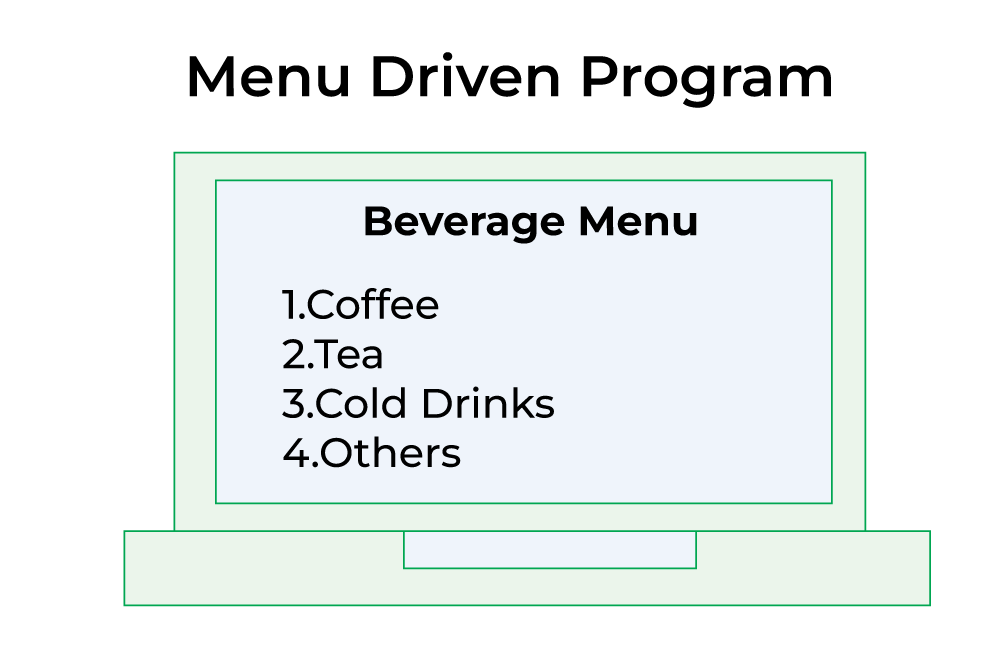Open In App

# Menu-Driven program using Switch-case in C

A Menu Driven program is a program that represents a menu of options to the user and different actions are performed based on different options. In this article, we will learn to write a Menu Driven program using Switch-case in C.## Menu Driven Program in C

The below program demonstrates an example of a Menu-Driven program using a Switch case to calculate:

1. Area of a circle
2. Area of square
3. Area of sphere

## C

 `// C program to illustrate Menu-Driven program using``// Switch-case``#include ` `// Function prototypes``int` `input();``void` `output(``float``);` `// driver code``int` `main()``{``    ``float` `result;``    ``int` `choice, num;` `    ``// printing menu``    ``printf``(``"Press 1 to calculate area of circle\n"``);``    ``printf``(``"Press 2 to calculate area of square\n"``);``    ``printf``(``"Press 3 to calculate area of sphere\n"``);``    ``printf``(``"Enter your choice:\n"``);` `    ``// taking input``    ``choice = input();` `    ``// switch statement to print output according to the``    ``// choice``    ``switch` `(choice) {``    ``case` `1: {``        ``printf``(``"Enter radius:\n"``);``        ``num = input();``        ``result = 3.14 * num * num;``        ``printf``(``"Area of sphere="``);``        ``output(result);``        ``break``;``    ``}``    ``case` `2: {``        ``printf``(``"Enter side of square:\n"``);``        ``num = input();``        ``result = num * num;``        ``printf``(``"Area of square="``);``        ``output(result);``        ``break``;``    ``}``    ``case` `3: {``        ``printf``(``"Enter radius:\n"``);``        ``num = input();``        ``result = 4 * (3.14 * num * num);``        ``printf``(``"Area of sphere="``);``        ``output(result);``        ``break``;``    ``}``    ``default``:``        ``printf``(``"wrong Input\n"``);``    ``}``    ``return` `0;``}` `// function to take input``int` `input()``{``    ``int` `number;``    ``scanf``(``"%d"``, &number);``    ``return` `(number);``}` `// function to print output``void` `output(``float` `number) { ``printf``(``"%f"``, number); }`

Output

```Press 1 to calculate area of circle
Press 2 to calculate area of square
Press 3 to calculate area of sphere